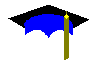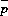JPL's Wireless Communication Reference Website

Chapter: Telephony Systems Also related to Chapter: Analog and Digital Transmission Also related to Chapter: Mobile Propagation Channels, Section: Fading, Rayleigh fading, probability densityThe mobile Rayleigh or Rician radio channel is characterized by rapidly changing channel characteristics. As typically a certain minimum (threshold) signal level is needed for acceptable communication performance, the received signal will experience periods of
• sufficient signal strength or "non-fade intervals"
• insufficient signal strength or "fades"
During fades the user experiences a signal outage.The signal outage probability is fairly simple to compute if one knows the probability distribution of the fading (e.g. Rayleigh or Rician / Nakagami) and outage occurs if the signal drops below the noise power level. The derivation involves an integration over the pdf of wanted and interfering signal power.The link budget calculation provides an estimate of the margin for how deep a signal fade can be, before the receiver looses the signal.The calculation becomes much more complicated if the (multiple) interfering signals also exhibit fading. Moreover shadowing affects the outage probability also.

Outage Probability versus Efficiency

A crucial aspect in the evaluation and planning of radio networks is the computation of the effect of co-channel interference in radio links. The amount of interference that can be tolerated determines the required separation distance between co-channel cells and therefore also the efficiency of the network. The link performance of cellular telephone networks was first studied around 1980 by Gosling, French and Cox. Initial analyses were limited to outage probabilities in continuous wave (CW) voice communication, taking into account path loss and flat (frequency non-selective) Rayleigh fading. In the 1980's the technique for computing outage probabilities was refined step by step, considering among other things shadowing, multiple interfering signals cumulating coherently or, more realistically, incoherently, the modulation technique and error correction method, and more recently the presence of a dominant line-of-sight propagation path, as it occurs in micro-cellular networks. The stochastic occupation of nearby co-channel cells according to the traffic laws by Erlang was included in some studies.

Computational Results

Any cellular operator will use topographic data bases to estimate outage probabilities in the area covered by the telephone service, but results for idealized hexagonal cell layouts are nonetheless illustrative for the effect of the reuse distance and shadowing.Figure: Signal outage probability versus normalized reuse distance for n = 1 ,.., 6 interfering signals. Shadowing 6 dB. Receiver threshold 10 dB. Plane earth loss (40 log d).

Evidently, the operator can reduce the outage probability by choosing larger reuse distances. However, this requires large cluster sizes, which results in poor spectrum efficiency.Figure: Outage probability versus degree of shadow fading for 1 and 6 interfering signals. Shadowing 6 dB. Receiver threshold 10 dB. Reuse distance equal 10 times the cell radius. Plane earth loss (40 log d).

For a crude propagation model that does not include terrain data, the standard deviation of the error is about 12 dB. In such case, either unacceptably large outage probabilities would be experienced or the cluster size would have to be unacceptable large. Hence, improved cell planning is required and virtually no operator plans its services considering idealized hexagonal cells. Mostly topographical data bases are used to estimate the area-mean signal power and interference powers as accurately as possible.

Computational Methods

Computation of the outage probability requires that we find the probability that the signal-to-interference ratio drops below a certain threshold. See: derivation. As all (fading) signals have fluctuating signal powers, this mean that we must integrate over the probability density functions of all signals involved.
• fading and shadowing of each interfering signal. Mostly it is assumed that
• interfering signals fade independently and independent of the wanted signal
• interfering signal powers accumulate incoherently, i.e., that is we may add powers.
In such case the pdf of the total interference power is the convolution of the pdf power of individual interfering signals
The number of interfering signals is six in a typical hexagonal reuse pattern, but interfering signals may use discontinuous voice transmission. In such case the terminal switches of the carrier during speech pauses to reduce the amount of interference caused to other users.Mathematical Background

In the special case that the wanted signal is Rayleigh fading, the received wanted signal is exponentially distributed. That implies that our integration can be rewritten as a Laplace transformation. The outage probability becomes

P(outage) = 1 - P(C/I > z) = 1 - Laplace(f ; z /)

where
• C/I is the signal-to-interference ratio
• z is the minimum required C/I ratio
• f is the pdf of total interference power
•is the local-mean power of the wanted signal
• Laplace(g ; s) is the Laplace image of function g, evaluated in the point s.Click here for a discussion of how to write a PASCAL program that computes the outage probability in a channel with Rayleigh fading and shadowing.

Generic Expressions

Similar expressions can be found for Rician and Nakagami fading wanted signals.
A generic expression exists that is suitable for rapid computer evaluation.

(Note: Use of the Nakagami or gamma distribution to approximate a Rician distribution appeared inaccurate and overly optimistic.)